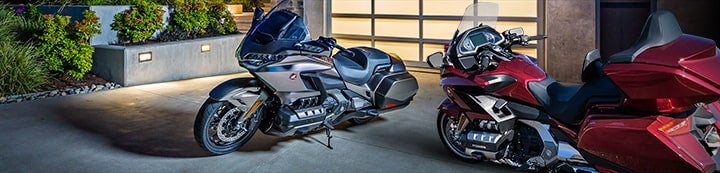1 - 4 of 4 Posts

#### Drair

·
##### Registered
Joined
·
711 Posts
Discussion Starter
I was looking at old recall notices for 1st generation GL1800s like the one I recently got, and found the following (attached). It shows an asterisk (*) where my VIN has a zero, and defines that as a "check digit". WTH? Does it mean that any bike's digit in that spot is covered by the recall, or that the bike's VIN has to be a specific digit to be covered? I don't have a dealer in town to ask. Besides, this recall is issued TO dealers, so shouldn't it be obvious which bikes it is supposed to pertain to? Maybe I'm just stupid tonight but every time I look at this it leaves me baffled.

#### Andy Cote

·
##### Registered
Joined
·
764 Posts
Don't worry about the asterisk. If your serial number (last eight digits) is in the range it's covered by the recall. Check digit is just a mathematical calculation based on the other digits to help prevent modifications, forgeries and theft.

•Art H., Ron Fuller, rayjoe and 2 others

#### Farmguy

·
##### Registered
Joined
·
7,560 Posts
Condensed explanation of VIN check-digit, from wikipedia: (article has a bunch more info about how it's calc'd)

Check-digit validation is compulsory for all road vehicles sold in North America.

When trying to validate a VIN with a check digit, first either (a) remove the check digit for the purpose of calculation or (b) use a weight of zero (see below) to cancel it out. The original value of the check digit is then compared with the calculated value. If the calculated value is 0–9, the check digit must match the calculated value. If the calculated value is 10, the check digit must be X. If the two values do not match (and there was no error in the calculation), then there is a mistake in the VIN. However, a match does not prove the VIN is correct, because there is still a 1/11 chance that any two distinct VINs have a matching check digit: for example, the valid VINs 5GZCZ43D13S812715 (correct with leading five) and SGZCZ43D13S812715 (incorrect with leading character "S").

There are lots of world wide 'rules' about meaning of the characters in a VIN.
First section is manufacturer, then characteristics of vehicle - In NA, the last number of this section is the 'check digit',
then model year code, plant code and then sequence code (serial number).

In the VIN's in the pic, the number after the check digit -- 2=2002, 3=2003...J=2018, K=2019 (and they don't use I, because it can be confused with 1)
1988 was also J, 1989 was K... the reuse sometimes causes confusion with owners/insurers.

A = 1980 L = 1990 Y = 2000 A = 2010 L = 2020 Y = 2030
B = 1981 M = 1991 1 = 2001 B = 2011 M = 2021 1 = 2031
C = 1982 N = 1992 2 = 2002 C = 2012 N = 2022 2 = 2032
D = 1983 P = 1993 3 = 2003 D = 2013 P = 2023 3 = 2033
E = 1984 R = 1994 4 = 2004 E = 2014 R = 2024 4 = 2034
F = 1985 S = 1995 5 = 2005 F = 2015 S = 2025 5 = 2035
G = 1986 T = 1996 6 = 2006 G = 2016 T = 2026 6 = 2036
H = 1987 V = 1997 7 = 2007 H = 2017 V = 2027 7 = 2037
J = 1988 W = 1998 8 = 2008 J = 2018 W = 2028 8 = 2038
K = 1989 X = 1999 9 = 2009 K = 2019 X = 2029 9 = 2039

1 - 4 of 4 Posts